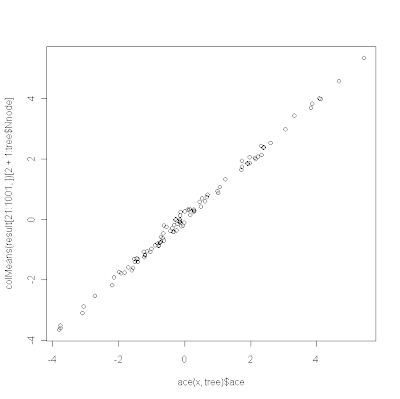## Thursday, November 24, 2011

### Bayesian ancestral character estimation

I've just been working on a new function for Bayesian ancestral character estimation (anc.Bayes). I'm still working out some of the bugs, but I have nonetheless posted a preliminary version online, here. (It also uses vcvPhylo(), which can be downloaded here).

1) If we take advantage of the multivariate normal density function dmnorm in the mnormt package, and my function vcvPhylo(), computing the likelihood (which we will use to calculate the posterior odds ratio) can be done in just a few lines of code:

C<-vcvPhylo(tree)
# function returns the log-likelihood
likelihood<-function(C,x,sig2,a,y){
logLik<-dmnorm(x=c(x,y),mean=rep(a,nrow(C)),varcov=sig2*C, log=TRUE)
return(logLik)
}

in which x is the data for species, y are the ancestral character values, a is the state at the root node, and sig2 is the evolutionary rate.

2. All the control parameters of the Bayesian MCMC (starting values, proposal distributions, and prior probabilities) are accessible to the user - but I have also tried to give the function the capacity to give reasonable values for these things if they are not provided by the user.

Although I'll warn again that this I am still working on this, it is not too soon to try it out. Let's simulate, run the MCMC, and then compare the results to ML estimates obtained using ace() in the ape package:

> require(phytools)
> source("vcvPhylo/vcvPhylo.R")
> source("anc.Bayes/anc.Bayes.R")
> set.seed(100)
> tree<-rtree(100)
> x<-fastBM(tree,sig2=2)
> result<-anc.Bayes(tree,x,ngen=100000)
Control parameters (set by user or default):
List of 7
\$ sig2   : num 2.03
\$ a      : num [1, 1] 0.00737
\$ y      : num [1:98] 0.00737 0.00737 0.00737 0.00737 0.00737 ...
\$ pr.mean: num [1:100] 1000 0 0 0 0 0 0 0 0 0 ...
\$ pr.var : num [1:100] 1e+06 1e+03 1e+03 1e+03 1e+03 1e+03 1e+03 1e+03 1e+03 1e+03 ...
\$ prop   : num [1:100] 0.142 0.142 0.142 0.142 0.142 ...
\$ sample : num 100
Starting MCMC...
Done MCMC.
> # plot the result against the MLEs
> plot(ace(x,tree)\$ace,colMeans(result[21:1001,])[2+1:tree\$Nnode])

Here, we get the following result:Obviously, I will post more on this as I improve it and I will also try to provide more guidance on MCMC control and priors.

1.2.